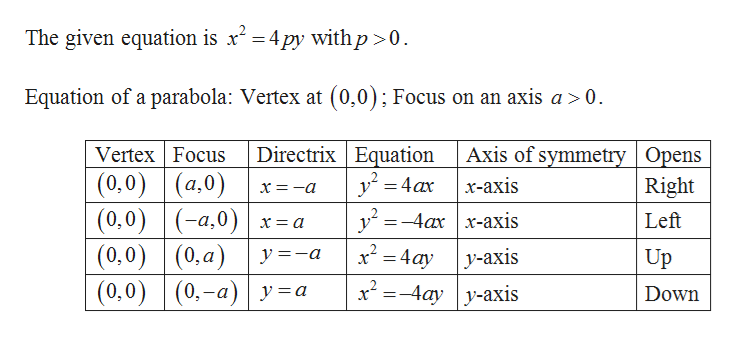# Given x2 = 4py with p> 0, the parabola opens (upward/downward/left/right).

Question
1 views

Given x2 = 4py with p> 0, the parabola opens (upward/downward/left/right).

check_circle

Step 1help_outlineImage TranscriptioncloseThe given equation is x' = 4 py with p>0. Equation of a parabola: Vertex at (0,0); Focus on an axis a> 0. Directrix Equation y = 4ax Axis of symmetry | Opens х-аxis Vertex Focus (0,0) (0,0) |(-a,0)| x= a (0,0) (0,a) (0,0) | (0.-a) | y=a (a,0) Right х — —а %3D -4ах | x-ахis Left х — а x² = 4ay y =-a y-axis Up =-4ay y-axis Down fullscreen

### Want to see the full answer?

See Solution

#### Want to see this answer and more?

Solutions are written by subject experts who are available 24/7. Questions are typically answered within 1 hour.*

See Solution
*Response times may vary by subject and question.
Tagged in

### Other